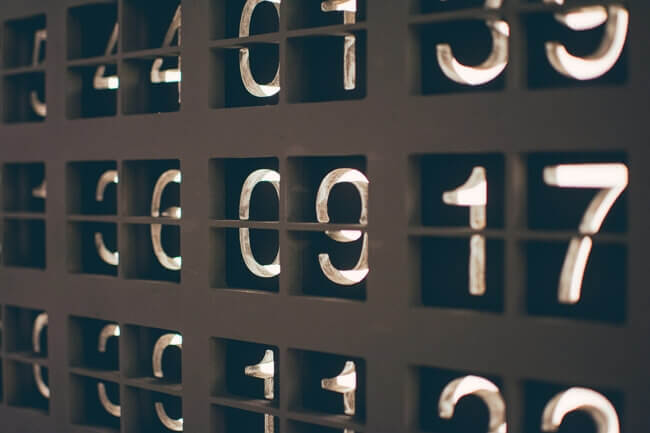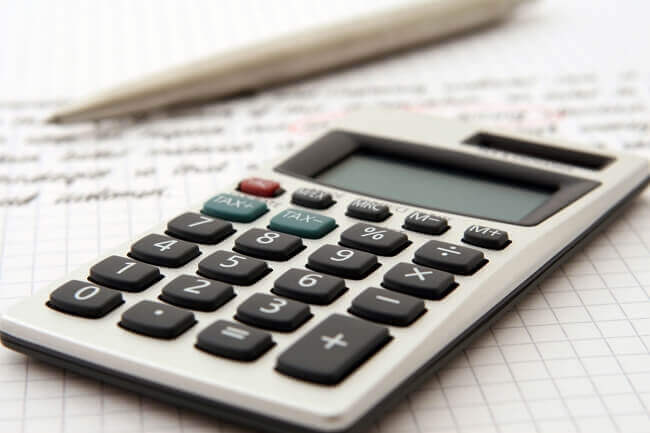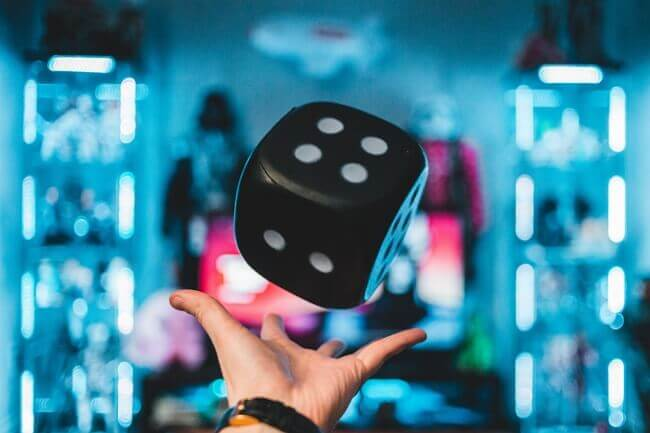# Odds Explained

## What are Odds?In statistics, the odds reflect the likelihood that the event will take place. In sports betting, the odds are the ratio of payoff to the stake and do not necessarily reflect exactly the probabilities.

Every time you place a bet with a bookmaker, you will be offered odds at the time, which means how much you can win. Betting odds allow you to calculate how much money you will win if you place a bet.

Understanding the meaning of betting odds is easy, but the most difficult part of this process is knowing whether the odds offered by bookmakers for a particular market reflect the exact probability. Only time and experience will help you identify if odds shown are higher than the probability of such event. If you believe the chance of a bet winning is higher than its implied probability, then you believe the bet is good value.

## How Bookmakers calculate oddsEvery bettor, every punter should know the real probabilities to make a profit, and then compare odds with the chances of the particular event. If it were so easy…

Let’s see an example of how it works.

We will believe that true probabilities for a football match are:

 Possible outcome Probability calculated by us Convert to Fractional Odds Convert to Decimal Odds Team A Wins ≅ 33 % chance 2/1 3.00 Draw ≅ 31 % chance 11/5 3.20 Team B Wins ≅ 36 % chance 9/5 2.80 TOTAL ≅ 100 %

But Bookmakers modify chances of winning:

 Possible outcome Probability calculated by Bookie Convert to Fractional Odds Convert to Decimal Odds Team A Wins ≅ 34 % chance 19/10 2.90 Draw ≅ 33 % chance 2/1 3.00 Team B Wins ≅ 38 % chance 13/8 2.63 TOTAL ≅ 105 %

Now, total chances sum 105%, which will guarantee Bookmakers a profit of 5%. This case would be perfect for the sportsbook if everyone bets according to the odds. However, bookmakers employ complex formulas to calculate adjustments to make to odds, depending on the actual money flow on each outcome of an event.

## Types of Odds

### Fractional Odds

Fractional odds are most often way of showing odds in the UK. As the name suggests, these odds are displayed as a fraction. To understand this kind of odds you can follow the next formula:

 Fractional Odds = Net Profit you will win / Stake you will bet

6/1 means you can win six units for every one unit staked.

### Decimal Odds

Decimal odds are more common in Europe. They represent the return you will receive for every pound invested, including your stake. If the decimal odds are 3.50, your total return will be £3.50 for every pound staked. Net profit will be £2.50 (£3.50-£1).

 Decimal Odds = Return for every pound invested, included your stake.

6/1  would be Decimal 7.

Betting £1 on Decimal 7 would return £7.

### American Odds

American Odds are also known as Moneyline or US Odds. Odds can be shown positive or negative.

 A positive number means how much profit you would win if you staked £100 stake.A negative number means how much stake you would place to win £100.

6/1 (Decimal 7) would be +600.

Betting £1 on American Odds -400 would return £1.25

### Convert Fractional Odds to Decimal Odds

There is no difference between decimal and fractional odds.

To convert 5/2 fractional odds to decimal odds: (5/2) + 1 = 3.5 decimal odds.

## Probability in Sports Betting

### DefinitionNow we want to see what those odds mean in terms of probability.

The implied probability is a conversion of odds into a percentage and eliminates the bookmaker edge to express true odds of an event occurring.

If you believe the chance of a bet winning is higher than its implied probability, then you believe the bet is good value.

### How to convert Fractional betting odds

If the fraction betting odds are 3/1, the formula would be:

 3/1 Odds ⇒ 1 / (3 + 1) = 1 / 4 = 0,25 x 100 = 25 % probability

### How to convert Decimal betting odds

If the decimal odds are 4, the formula would be:

 4.00 Odds ⇒ 1 /4= 0,25 x 100 = 25 % probability

### How to convert American betting odds

If the American odds are +300, the formula would be:

 +300 Odds ⇒ 100 /(100+300)= 100/400= 0,25 x 100 = 25 % probability